Ex 9.2

Chapter 9 Class 11 Sequences and Series (Term 1)
Serial order wise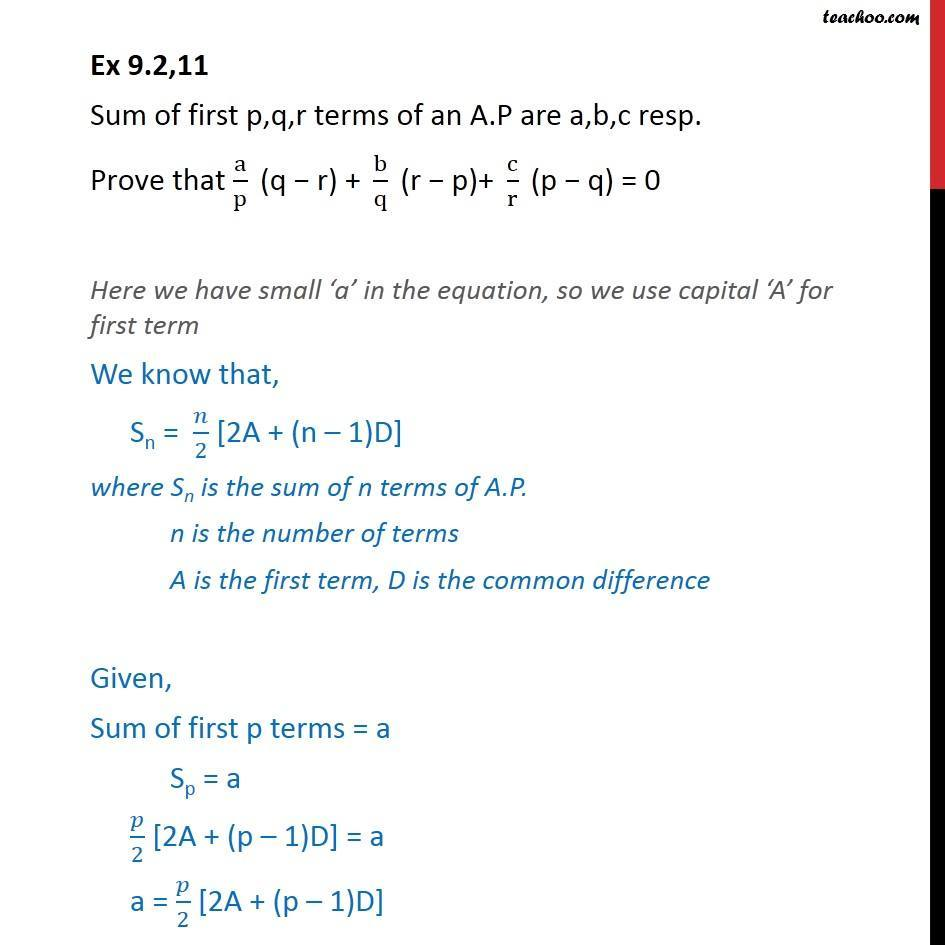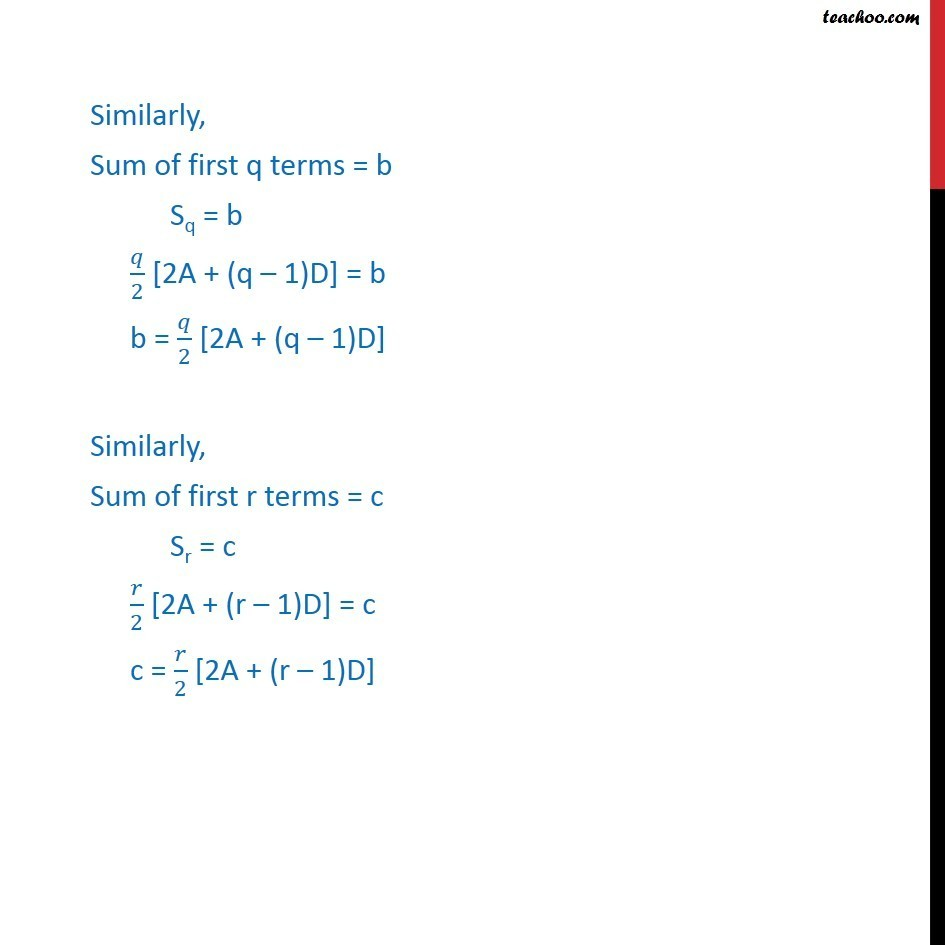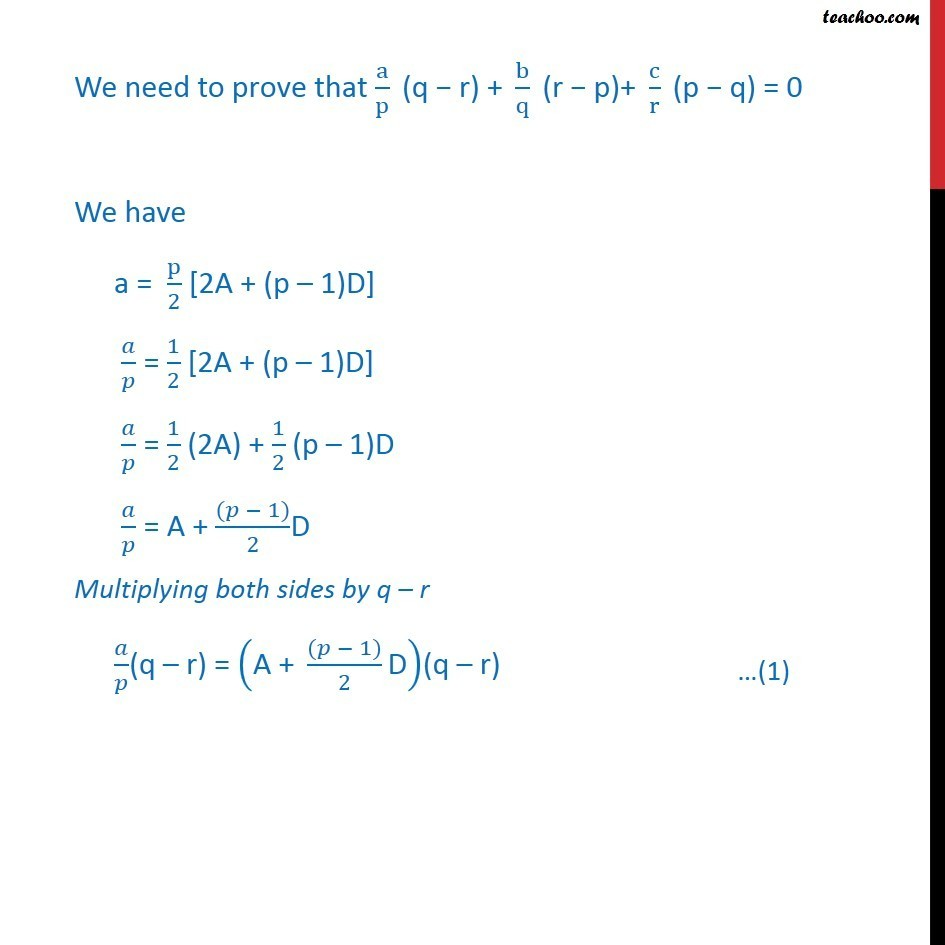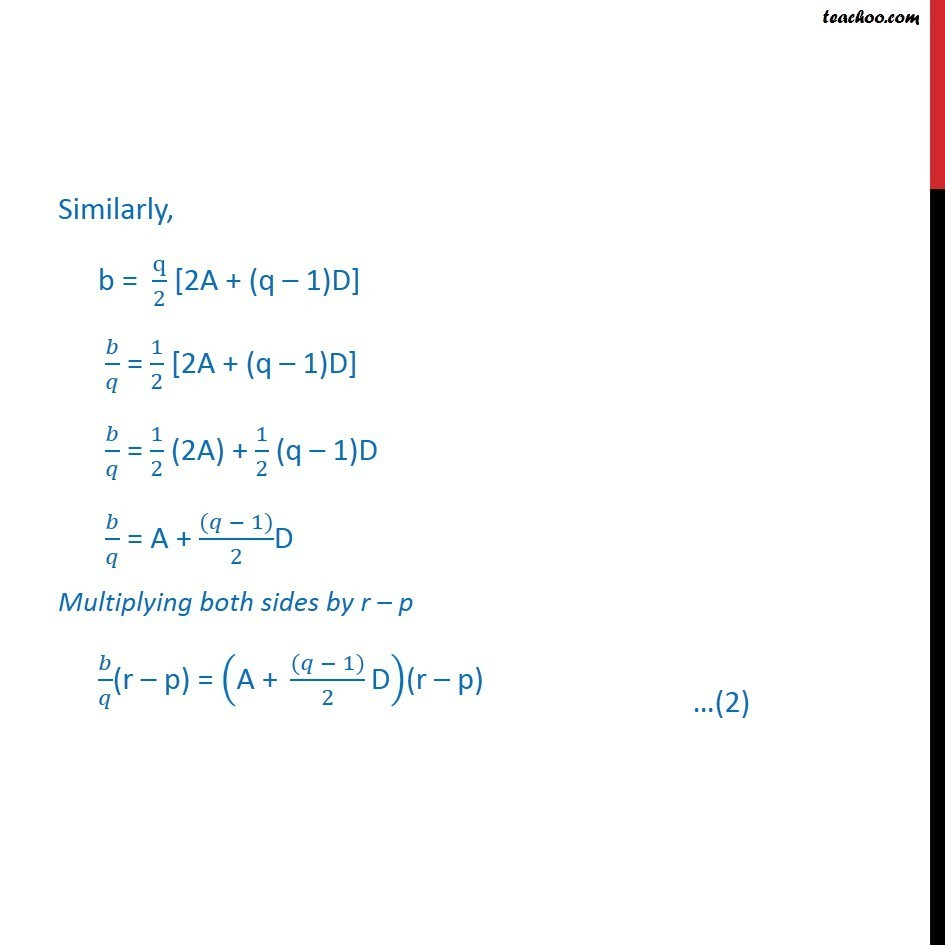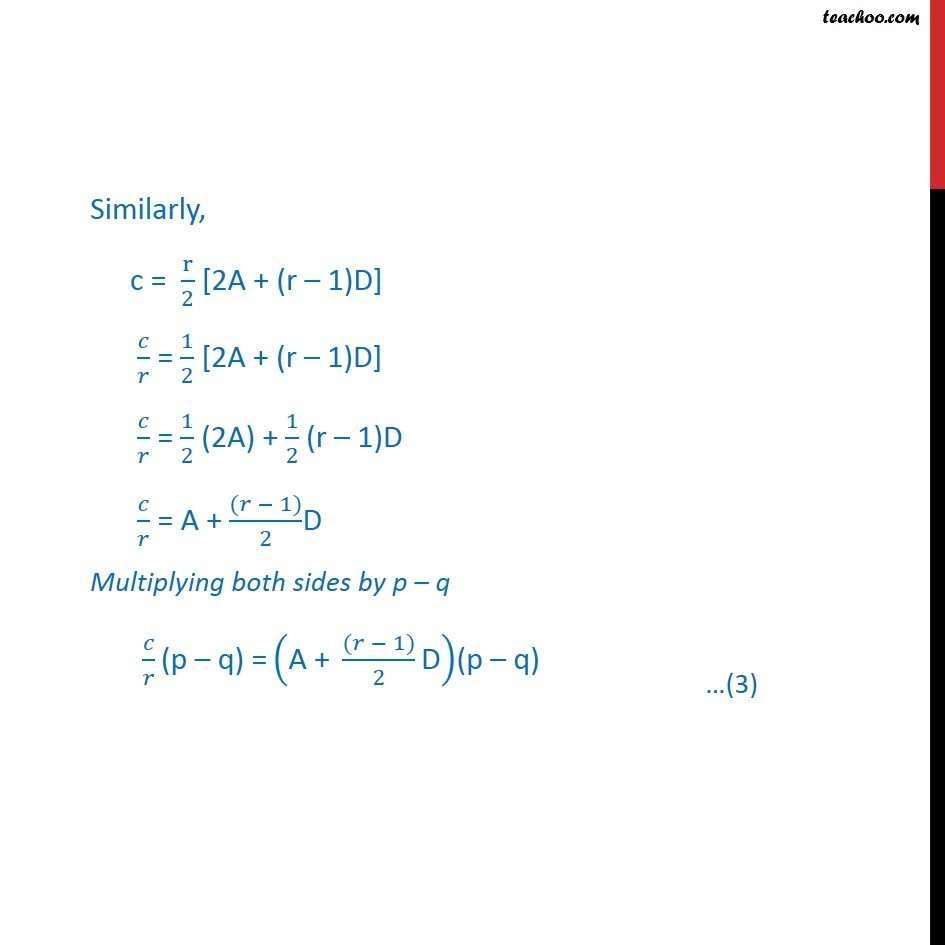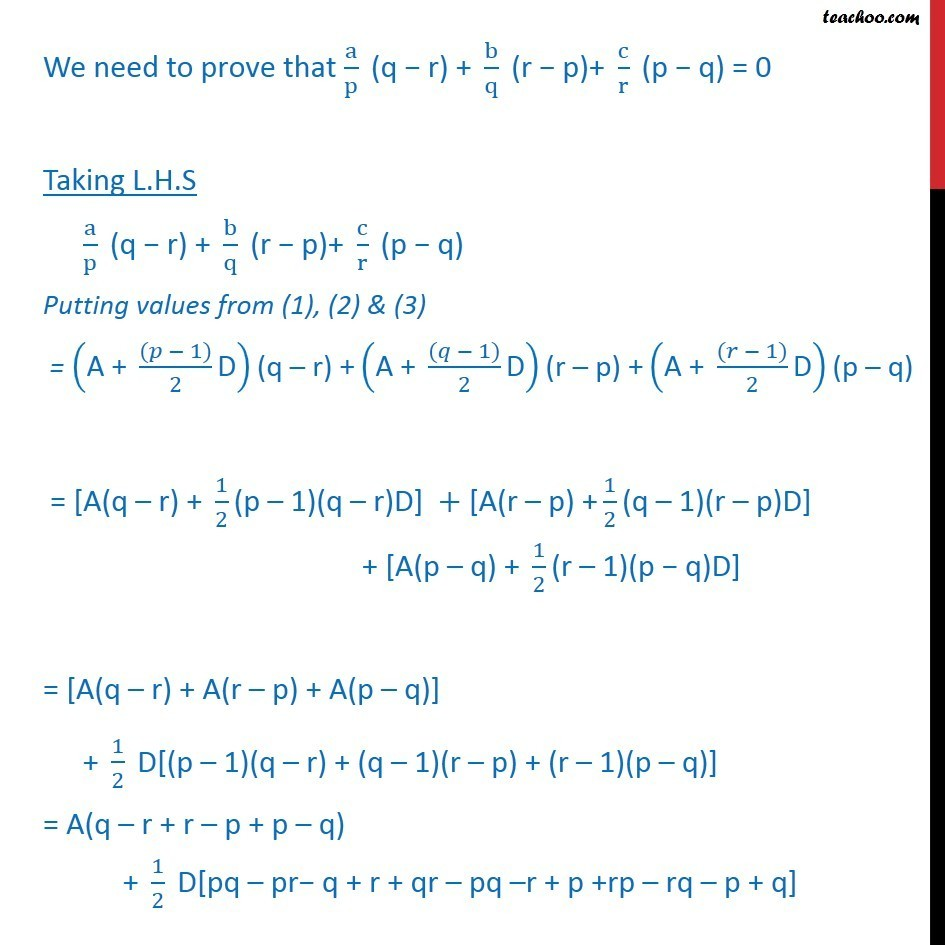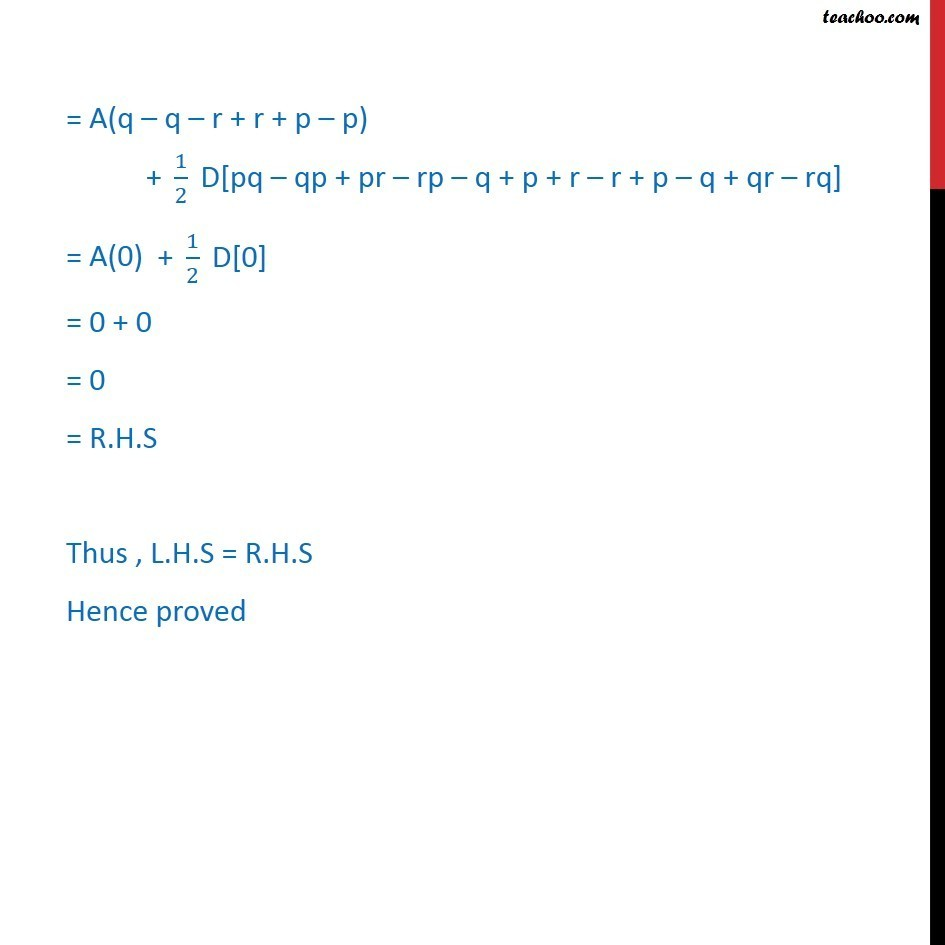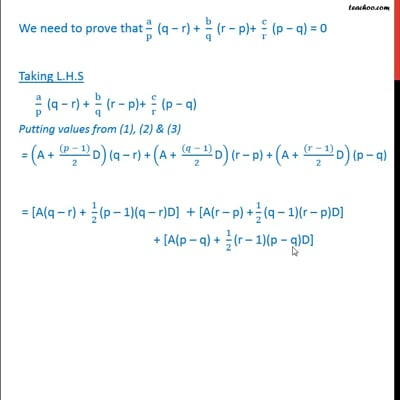This video is only available for Teachoo black users

### Transcript

Ex 9.2,11 Sum of first p,q,r terms of an A.P are a,b,c resp. "Prove that" a/p " (q r) + " b/q " (r p)+ " c/r " (p q) = 0" Here we have small a in the equation, so we use capital A for first term We know that, Sn = /2 [2A + (n 1)D] where Sn is the sum of n terms of A.P. n is the number of terms A is the first term, D is the common difference Given, Sum of first p terms = a Sp = a /2 [2A + (p 1)D] = a a = /2 [2A + (p 1)D] Similarly, Sum of first q terms = b Sq = b /2 [2A + (q 1)D] = b b = /2 [2A + (q 1)D] Similarly, Sum of first r terms = c Sr = c /2 [2A + (r 1)D] = c c = /2 [2A + (r 1)D] We need to "prove that" a/p " (q r) + " b/q " (r p)+ " c/r " (p q) = 0" We have a = p/2 [2A + (p 1)D] / = 1/2 [2A + (p 1)D] / = 1/2 (2A) + 1/2 (p 1)D / = A + (( 1))/2D Multiplying both sides by q r / (q r) = ("A + " (( 1))/2 "D" )(q r) Similarly, b = q/2 [2A + (q 1)D] / = 1/2 [2A + (q 1)D] / = 1/2 (2A) + 1/2 (q 1)D / = A + (( 1))/2D Multiplying both sides by r p / (r p) = ("A + " (( 1))/2 "D" )(r p) Similarly, c = r/2 [2A + (r 1)D] / = 1/2 [2A + (r 1)D] / = 1/2 (2A) + 1/2 (r 1)D / = A + (( 1))/2D Multiplying both sides by p q " " / "(p q) =" ("A + " (( 1))/2 "D" )(p q) We need to "prove that" a/p " (q r) + " b/q " (r p)+ " c/r " (p q) = 0" Taking L.H.S a/p " (q r) + " b/q " (r p)+ " c/r " (p q)" Putting values from (1), (2) & (3) = ("A + " (( 1))/2 "D" )"(q r) +" ("A + " (( 1))/2 "D" )"(r p) +" ("A + " (( 1))/2 "D" )"(p q)" = "[A(q r) + " 1/2 "(p 1)(q r)D]" +"[A(r p) +" 1/2 "(q 1)(r p)D]" "+ [A(p q) + " 1/2 "(r 1)(p q)D]" = "[A(q r) + A(r p) + A(p q)]" (" " @"+ " 1/2 " D[(p 1)(q r) + (q 1)(r p) + (r 1)(p q)] " ) = "A(q r + r p + p q)" "+ " 1/2 " D[pq pr q + r + qr pq r + p +rp rq p + q]" = ("A(q q r + r + p p) " ) "+ " 1/2 " D[pq qp + pr rp q + p + r r + p q + qr rq]" = ("A(0) " ) "+ " 1/2 " D" = 0 + 0 = 0 = R.H.S Thus , L.H.S = R.H.S Hence proved